Next: Beyond Local Optimization: Annealing Up: Optimization Examples Previous: Optimization Examples

## Fourier Decomposition Optimization

Let us now consider an arbitrary function represented by its Fourier decomposition. This function, denoted F(x), is a linear combination of sinusoids as in Equation 6.22.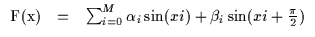(6.22)

Since we have a way to solve for the quadratic bound for a canonical sinusoid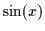at a contact point z, we should be able to generalize this bound to all the individual terms in Equation 6.22 using some bound properties such as Equation 6.15. Each sinusoid in the linear combination in Equation 6.22 is of the form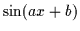with a>06.3. In addition, we need to modify the contact point zwith which we will look up the values of w, k and y since these computations are particular toand not.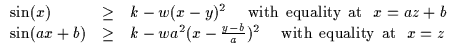(6.23)

Figure 6.9(a) depicts the function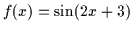(a=2, b=3) which we wish to bound with a contact point at x*=z=-6. We instead compute the parabolic bound forat x=(2*(-6)+3)=-9 and obtain w=0.23, y=-10.98 and k=0.49. We can thus insert those quantities into the parabola expression and obtain the bound for the desired function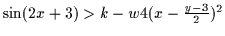which is plotted in Figure 6.9 (c).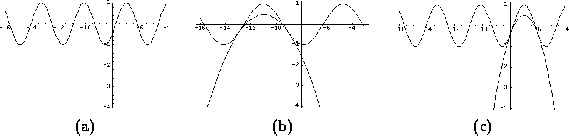Using Equation 6.14 we can further manipulate each term by scaling it appropriately. Thus, we can get an expression for the lower bound on F(x) in terms of a multitude of parabolic elements (p2i(x) and p2i+1(x)).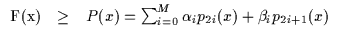(6.24)

Since it is necessary to now maximize the above sum of bounds, we can merely do so by taking the derivative of the sum with respect to xand setting that to 0. Maximizing the sum of quadratic terms thus merely reduces to the solution of a linear system. This is not quite as trivial as choosing the y locus as the maximum for a single parabola but it is still extremely efficient. This operation is depicted in Equation 6.25. The x value computed is the locus of the maximum of the parabola. Thus, the equation implements one maximization step and yields the operating or contact point for the next iteration (for the bounding step). Repeating these two steps will converge to a local maximum of the function as in Figure 6.10.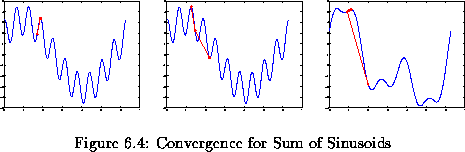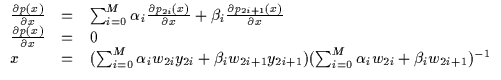(6.25)

Since we are able to optimize any combination of sinusoids with the above, and any arbitrary (l2-bounded) analytic function can be approximated by sinusoids (even in multiple dimensions), it is possible in principle to use the above derivation to optimize a wide variety of functions. These functions could also be non-analytic functions which are only sampled and approximated by sinusoids using least-squares. In addition, the above derivations for the canonical sinusoid sin(x) function could have been done for another function such as radial basis functions, exp(x), and so on and then generalized to linear combinations of this canonical functions. One small caveat exists when using linear combinations of arbitrary bounded functions: the negative of the function is bounded differently from the function itself. Thus, for arbitrary linear combinations, one needs to have a bound for canonical f(x) and -f(x) (since a bound flips from lower to upper bound due to the negative sign).Next: Beyond Local Optimization: Annealing Up: Optimization Examples Previous: Optimization Examples
Tony Jebara
1999-09-15